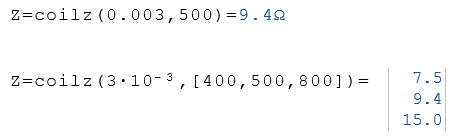# Coilz Function

Calculates the impedance for the specified coil and frequency

## Calculate the impedance

The Coilz function calculates the impedance for the specified coil and frequency. The parameters for the coil L and the frequency f can be real numbers or lists of real numbers.

Coilz (L, f)

### Legend

 L Inductance in Henry f Frequency in Hz

### Example